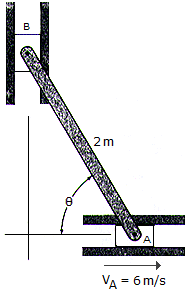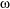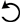# Engineering Mechanics - Planar Kinematics of a Rigid Body (PKRB) - Discussion

### Discussion :: Planar Kinematics of a Rigid Body (PKRB) - General Questions (Q.No.2)

2.The 2-m-long bar is confined to move in the horizontal and vertical slots A and B. If the velocity of the slider block at A is 6 m/s, determine the bar's angular velocity and the velocity of block B at the instant= 60°.

 [A].AB = 3.46 rad/s, vB = 3.46 m/s 9 [B].AB = 3.00 rad/s, vB = 3.00 m/s 9 [C].AB = 3.00 rad/s, vB = 6.00 m/s 9 [D].AB = 6.00 rad/s, vB = 10.39 m/s 9

Explanation:

No answer description available for this question.

 Arpit Lasod said: (Jan 4, 2011) consider rod AB, and A as I.C.R.(instantenous center of rotation) Va=6m/s r=2m(length of the rod) Va=rw w=3rad/s consider rod AB, and B as I.C.R.(instantenous center of rotation) r=2m w=3rad/s Vb=rw Vb=6m/s

 Rios said: (Jan 31, 2018) Doing a triangle, the displacement is equal to 2 sin(60). VA= wr. 6= w(2sin(60)). w=3.46.

 Jani Dhruvkumar said: (Aug 3, 2019) No, the Correct one is; Hence both points are on single link AB, therefore, the value of w=wa=wb. Now, W=(Va/Ia)=(Vb/Ib). (Va=6m/s). (Ia=√3 and Ib=1 from instantaneous centre method). Therefore wb=wa=w=3.46.

 Pankaj said: (Apr 26, 2021) Vb - Va=Vba. Vba = wrba. Vb - va = w rba. Vb = 6i + w*2(-cos60i +sin60j). Vb is in y direction so we only take y component and it's x component should be zero Vb = 2wsin60, 6-2wcos60=0. w = 6/(2cos60). w = 6, Vb = 2 * 6 * √3/2. Vb = 6√3, Vb = 10.39.